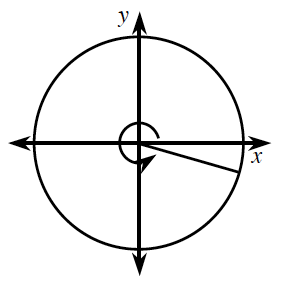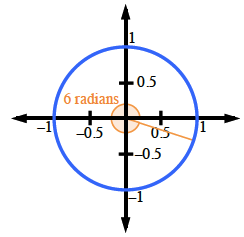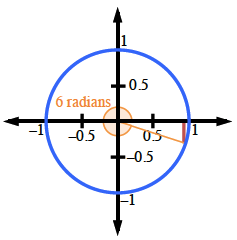### Home > CCA2 > Chapter 7 > Lesson 7.1.6 > Problem7-93

7-93.

Draw a picture of an angle that measures $6$ radians.1. Approximately how many degrees is this?

Notice that $2π$ radians $≈ 2(3.14) ≈ 6.27$.So it's a little less than $360^\circ$.

$\frac{6}{2 \pi} = \frac{x}{360}$

$6\ \text{radians}\ \approx344^{\circ}$

$\sin(6)=−0.3$